Author: Oscar Cronquist Article last updated on September 14, 2021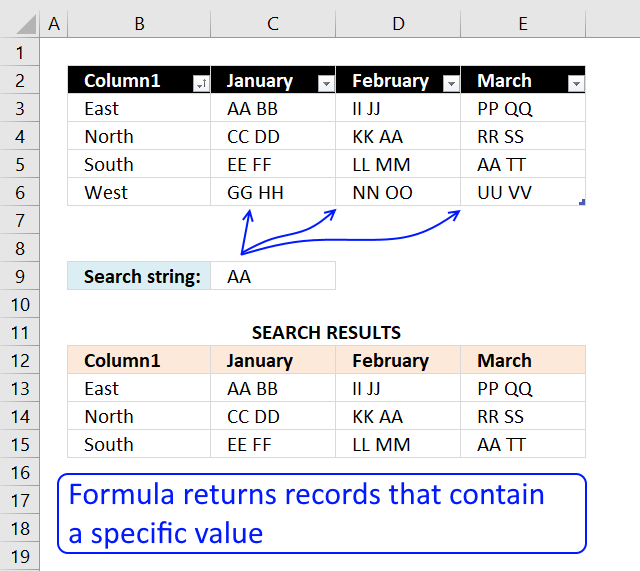This article explains different techniques that filter rows/records that contain a given text string in any of the cell values in a record. To filter records based on a condition read this: VLOOKUP - Extract multiple records based on a condition, that article also demonstrates how to filter records using the new FILTER function only available in Excel 365.

Example, the image above shows the data in cell range B3:E6, the condition is in cell C9. The formula extracts records from B3:E6 if at least one cell contains the string.

Row 3 is extracted above because string AA is found in cell C3. Row 4 and 5 are also extracted because string AA is found in cell D4 and E5 respectively. Row 6 is not extracted, there is no cell containing the search string.

Read section 1.1 for a detailed explanation of how this formula works. I have built a formula that matches two criteria and return multiple records.

## 1. Search for a text string in a data set and return multiple records [Array formula]This example demonstrates a formula that extracts records if any cell on the same row contains a specific value specified in cell C9.

This means also that the formula returns the same record multiple times if multiple cells contain the search value.

Array formula in B13:

=IFERROR(INDEX(Table1, SMALL(IF(ISNUMBER(FIND(\$C\$9, Table1)), MATCH(ROW(Table1), ROW(Table1)), ""), ROWS(\$A\$1:A1)), COLUMNS(\$A\$1:A1)), "")

To enter an array formula, type the formula in cell B13 then press and hold CTRL + SHIFT simultaneously, now press Enter once. Release all keys.

The formula bar now shows the formula with a beginning and ending curly bracket telling you that you entered the formula successfully. Don't enter the curly brackets yourself.

Copy cell B13 and paste to cell range B13:E16. Replace FIND function with SEARCH function if you don't want the formula to perform a case-sensitive search.

#### Search for a text string in a data set and return multiple records (no duplicates)

Array formula in cell B13:

=IFERROR(INDEX(Table1, SMALL(IF(MMULT(ISNUMBER(FIND(\$C\$9, Table1))*1, ROW(Table1)^0), MATCH(ROW(Table1), ROW(Table1)), ""), ROWS(\$A\$1:A1)), COLUMNS(\$A\$1:A1)), "")

The array formula above returns unique distinct records meaning no duplicate records if more than one cell matches the search string.

### 1.1 Explaining array formula in cell B13

#### Step 1 - Identify cells containing the search string

The FIND function returns the starting point of one text string within another text string, it returns a number representing the position of the found string. If not found the function returns the #VALUE! error.

FIND(\$C\$9, \$B\$3:\$E\$6)

returns

{#VALUE!, 1, #VALUE!, #VALUE!; #VALUE!, #VALUE!, 4, #VALUE!; #VALUE!, #VALUE!, #VALUE!, 1; #VALUE!, #VALUE!, #VALUE!, #VALUE!}

The ISNUMBER function returns TRUE if the value in the array is a number and FALSE if not a number, it returns FALSE even if the value is an error value which is handy in this case.

ISNUMBER(FIND(\$C\$9, \$B\$3:\$E\$6)) creates this array displayed in cell B11:E14: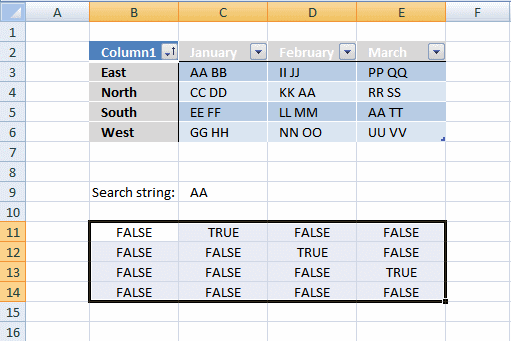Column B has no cells containing string "AA".

Column C has 1 cell containing string "AA". Cell C3

Column D has 1 cell containing string "AA". Cell D4.

Column E has 1 cell containing string "AA". Cell E5.

#### Step 2 - Return row number

We need to calculate the row number for each cell in order to replace TRUE in the array with the corresponding row number. To create the array we need we use the MATCH function and the ROW function.

MATCH(ROW(Table1), ROW(Table1)) returns this array displayed in cell range B11:E14: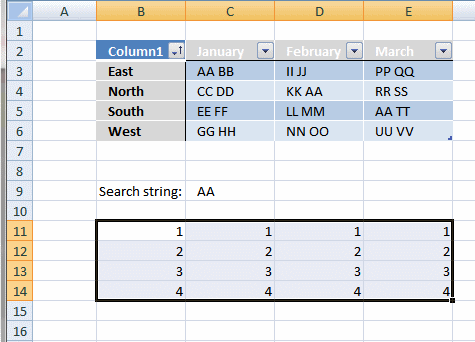If string "AA" is found in a cell in the table the corresponding row number is returned.

#### Step 3 - Replace boolean values with row numbers

The IF function has three arguments, the first one must be a logical expression. If the expression evaluates to TRUE then one thing happens (argument 2) and if FALSE another thing happens (argument 3).

IF(ISNUMBER(FIND(\$C\$9, Table1)), MATCH(ROW(Table1), ROW(Table1)), "")

becomes

IF({#VALUE!, 1, #VALUE!, #VALUE!; #VALUE!, #VALUE!, 4, #VALUE!; #VALUE!, #VALUE!, #VALUE!, 1; #VALUE!, #VALUE!, #VALUE!, #VALUE!}, MATCH(ROW(Table1), ROW(Table1)), "")

becomes

IF({#VALUE!, 1, #VALUE!, #VALUE!; #VALUE!, #VALUE!, 4, #VALUE!; #VALUE!, #VALUE!, #VALUE!, 1; #VALUE!, #VALUE!, #VALUE!, #VALUE!}, {1; 2; 3; 4}, "")

and returns the following array shown in cell range B11:E14: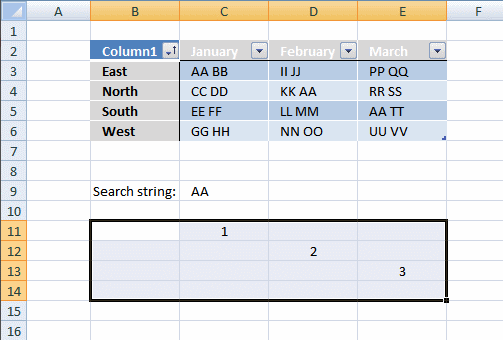#### Step 4 - Sort the row numbers from smallest to largest

To be able to return a new value in a cell each I use the SMALL function to filter column numbers from smallest to largest.

The ROWS function keeps track of the numbers based on an expanding cell reference. It will expand as the formula is copied to the cells below.

SMALL(IF(ISNUMBER(FIND(\$C\$9, Table1)), MATCH(ROW(Table1), ROW(Table1)), ""), ROWS(\$A\$1:A1))

returns 1.

#### Step 5 - Return a value at the intersection of a particular row and column

The INDEX function returns a value based on a cell reference and column/row numbers.

INDEX(Table1, SMALL(IF(ISNUMBER(FIND(\$C\$9, Table1)), MATCH(ROW(Table1), ROW(Table1)), ""), ROWS(\$A\$1:A1)), COLUMNS(\$A\$1:A1))

becomes

INDEX(Table1, 1, COLUMNS(\$A\$1:A1))

becomes

INDEX(Table1, 1, 1)

and returns "East" in cell B13.

#### Step 6 - Remove errors

The IFERROR function allows you to display a blank if the formula returns an error.

Note, the IFERROR function handles all errors. Use with caution.

## 2. Search for a text string in a data set and return multiple records [Excel 365]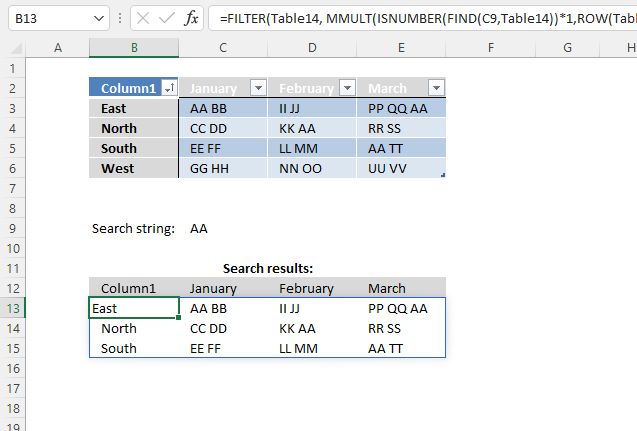The image above demonstrates an Excel 365 formula that extracts records based on a condition. The record is extracted if any cell in a record contains the condition.

Dynamic array formula in cell B13:

=FILTER(Table1, MMULT(ISNUMBER(FIND(C9, Table1))*1, ROW(Table1)^0))

This formula works only in Excel 365, it returns an array of values that spills to cells below and to the right automatically. The formula contains the new FILTER function.

### 2.1 Explaining formula in cell B13

#### Step 1 - Identify cells containing the given search string

The FIND function returns the position of a specific string in another string, reading left to right. Note, the FIND function is case-sensitive.

FIND(C9, Table1)

becomes

FIND("AA", {"East", "AA BB", "II JJ", "PP QQ AA";"North", "CC DD", "KK AA", "RR SS";"South", "EE FF", "LL MM", "AA TT";"West", "GG HH", "NN OO", "UU VV"}))

and returns

{#VALUE!, 1, #VALUE!, 7;#VALUE!, #VALUE!, 4, #VALUE!;#VALUE!, #VALUE!, #VALUE!, 1;#VALUE!, #VALUE!, #VALUE!, #VALUE!}.

The FIND function returns a #VALUE! error if no string is found.

#### Step 2 - Check if value in array is a number

The ISNUMBER function returns a boolean value TRUE or FALSE. TRUE if the value is a number and FALSE for anything else, also an error value.

ISNUMBER(FIND(C9, Table1))

becomes

ISNUMBER({#VALUE!, 1, #VALUE!, 7;#VALUE!, #VALUE!, 4, #VALUE!;#VALUE!, #VALUE!, #VALUE!, 1;#VALUE!, #VALUE!, #VALUE!, #VALUE!})

and returns {FALSE, TRUE, FALSE, TRUE;FALSE, FALSE, TRUE, FALSE;FALSE, FALSE, FALSE, TRUE;FALSE, FALSE, FALSE, FALSE}.

#### Step 3 - Convert boolean values to numerical equivalents

The asterisk lets you multiply a value or array, this action converts boolean values to numbers automatically. This step is required because the MMULT function can't work with boolean values.

ISNUMBER(FIND(C9, Table1))*1

becomes

{FALSE, TRUE, FALSE, TRUE;FALSE, FALSE, TRUE, FALSE;FALSE, FALSE, FALSE, TRUE;FALSE, FALSE, FALSE, FALSE} * 1

and returns

{0, 1, 0, 1; 0, 0, 1, 0; 0, 0, 0, 1; 0, 0, 0, 0}.

#### Step 4 - Create a number sequence

The ROW function calculates the row number of a cell reference.

ROW(ref)

ROW(Table1)

returns {3; 4; 5; 6}.

#### Step 5 - Change numbers to 1

The power of or exponent character is able to convert each number if number to the power of zero is calculated.

ROW(Table1)^0

becomes

{3; 4; 5; 6}^0

and returns {1; 1; 1; 1}.

#### Step 6 - Consolidate numbers

The MMULT function calculates the matrix product of two arrays, an array as the same number of rows as array1 and columns as array2.

MMULT(array1array2)

MMULT(ISNUMBER(FIND(C9, Table1))*1, ROW(Table1)^0)

becomes

MMULT({0, 1, 0, 1; 0, 0, 1, 0; 0, 0, 0, 1; 0, 0, 0, 0}, {1; 1; 1; 1})

and returns {2; 1; 1; 0}.

#### Step 7 - Filter values based on array

The FILTER function lets you extract values/rows based on a condition or criteria.

FILTER(arrayinclude, [if_empty])

FILTER(Table1, MMULT(ISNUMBER(FIND(C9, Table1))*1, ROW(Table1)^0))

becomes

FILTER(Table1, {2; 1; 1; 0})

and returns the following array in cell B13:

{"East", "AA BB", "II JJ", "PP QQ AA"; "North", "CC DD", "KK AA", "RR SS"; "South", "EE FF", "LL MM", "AA TT"}## 3. Search for a text string in a data set and return multiple records - Excel Table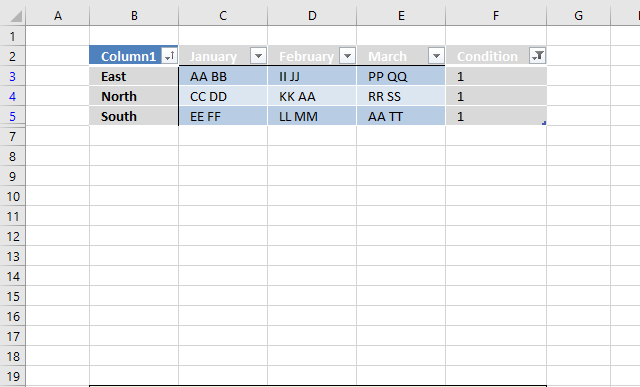This example demonstrates how to filter records if any of the cells on a row contains a specific string using an Excel Table. You need a formula and a helper column to accomplish this task.

You can't do this using the "Custom Autofilter" built-in to the Excel Table, there is no way to use OR logic between filters across columns, you need a formula to do this. As far as I know.

### 3.1 Convert dataset to an Excel defined Table

1. Select any cell within the dataset.
2. Press CTRL + T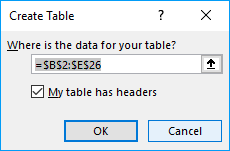3. Press with left mouse button on checkbox if your dataset contains headers for each column.
4. Press with left mouse button on OK button.

### 3.2 Add formula to Excel defined Table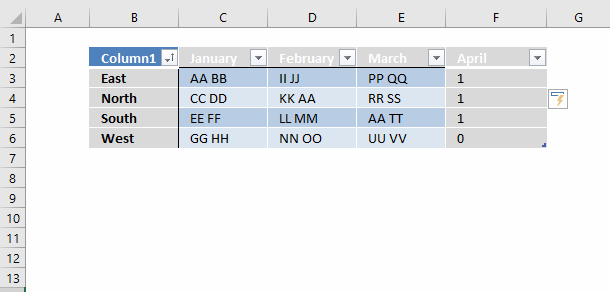1. Select cell F3.
2. Type formula: =COUNTIF(Table13[@[January]:[March]],"*AA*")
3. Press Enter.

Excel fills the remaining cells in the table for you and creates a header name for your new column automatically.

### 3.3 Explaining formula in cell F3

#### Step 1 - COUNTIF function

The COUNTIF function calculates the number of cells that is equal to a condition.

COUNTIF(rangecriteria)

#### Step 2 - Populate arguments

COUNTIF(Table13[@[January]:[March]],"*AA*")

range - Table13[@[January]:[March]] is a structured reference to data in columns January to March in table Table13. The at sign @ before the header names indicate that the reference is to values on the same row.

criteria - "*AA*" The asterisk character is a wildcard character that matches 0 (zero) to any number of characters. When we use a leading and trailing asterisk the criteria matches cells that contain "AA".

#### Step 3 - Evaluate formula

COUNTIF(Table13[@[January]:[March]],"*AA*")

becomes

COUNTIF({"AA BB", "II JJ", "PP QQ"}, "*AA*")

and returns 1 in cell F3. String AA was found once in cell range C3:E3. Note that the formula evaluates only cells on the same row, this is why the formula doesn't return an array of values.

### 3.4 Filter Excel Table

To filter the records containing string AA at least once follow these steps:

1. Press with left mouse button on black arrow next to the header name "April".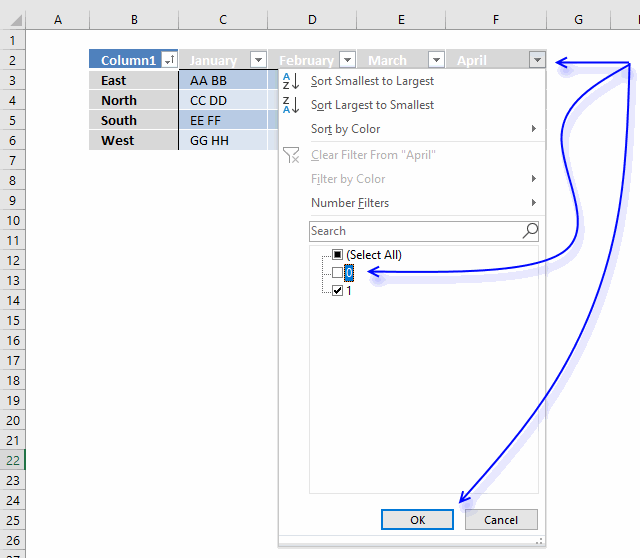2. Press with left mouse button on checkbox next to 0 (zero) to deselect it.
3. Press with left mouse button on OK button.April is not the correct header name, I changed it to Condition.

## 4. Search for a text string in a data set and return multiple records [Advanced Filter]The Advanced Filter is a powerful feature in Excel that allows you to perform OR-logic between columns. The asterisk lets you do a wildcard lookup meaning that a record is filtered if the text string is found somewhere in the cell value.1. Copy column headers and paste to cells above or below the dataset. Note, if you place them next to the dataset they may become hidden when the filter is applied.
2. Type the search condition and add an asterisk before and after the text string.
3. Add another search condition, make sure they are in a row each in order to perform OR-logic.
4. Repeat with the remaining criteria.

### 4.2 Apply filter

1. Select the dataset.
2. Go to tab "Data" on the ribbon.
3. Press with left mouse button on the "Advanced" button.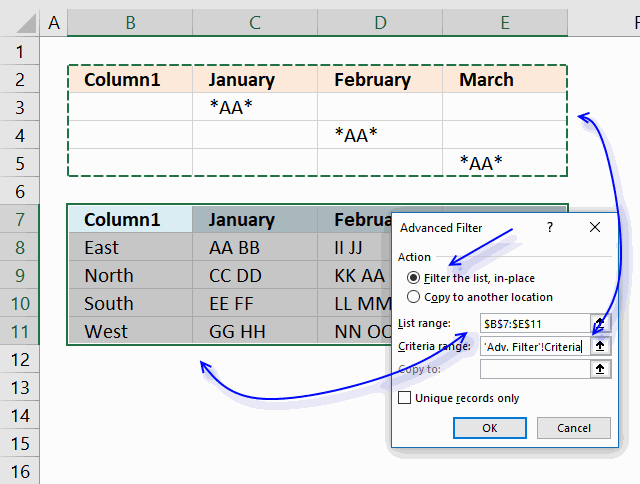4. Press with left mouse button on the radio button "Filter the list, in-place".
5. Select Criteria range:"
6. Press with left mouse button on "OK button.To delete the filter applied simply select a cell within the filtered dataset, then go to tab "Data" on the ribbon and press with left mouse button on "Clear" button.

## 5. Excel file

### Get the Excel filesearch-for-a-string-in-an-excel-table3.xlsx

### How do I extract rows that contain a string in a data set or table?

You can use a formula to extract records based on a search value, it also returns multiple records if there are any that match. The advantage of using a formula is that it is dynamic meaning the result changes as soon as a new search value is entered. The downside with the formula is that it may become slow if you have lots of data to work with.

### How do I filter rows that contain a string using Advanced Filter?

You also have the option to filter records using an Advanced Filter, it allows you to perform multiple search values using OR-logic across multiple columns. This article explains how to set it up, jeep in mind that it needs a small amount of manual work in order to apply new filters.

### How do I filter rows that contain a string using an Excel defined Table?

The Excel defined Table needs the COUNTIF function to accomplish the task which may slow down the calculation considerably if your data set is huge. I recommend using the Advanced Filter if speed is an issue.

The following article shows you how to VLOOKUP and return multiple values, the lookup value must match the entire cell value.

Recommended articles

5 easy ways to VLOOKUP and return multiple values
This post explains how to lookup a value and return multiple values. No array formula required.

Search for a cell value in a dataset:

Recommended articles

Search for a cell value in a dataset
Question: How do I identify rows that have a specific cell value in an Excel defined table? Array formula in […]

How to look for values in a column that contain two text strings:

Recommended articles

Partial match for multiple strings – AND logic
This article demonstrates formulas that let you perform partial matches based on multiple strings and return those strings if all […]

Search two columns for two text strings. The strings can be anywhere in these two columns but both text strings have to be found in different cell values or a single cell value and be on the same row to match:

Recommended articles

Partial match based on two conditions
Question: I want to search two columns with two search strings? The strings can be anywhere in these two columns […]

Search for three text strings in three columns, one text string in each column. Return values in which all three cases are found:

Recommended articles

Perform multiple partial matches and return records – AND logic
Question: Can expand this equation set into more than two colums of data, say if I had a first, middle […]

Search for multiple text strings in multiple columns, one text string in each column. Return values in which all text strings match:

Recommended articles

Extract records where all criteria match if not empty
Question: I second G's question: can this be done for more than 3? i.e. (Instead of last name, middle, first) […]

Search for a single text string in a single column and return multiple matches.

Recommended articles

Partial match and return multiple adjacent values
This article demonstrates array formulas that search for cell values containing a search string and returns corresponding values on the […]

Search for a text string in a single column and return multiple corresponding values.

Recommended articles

Partial match and return multiple adjacent values
This article demonstrates array formulas that search for cell values containing a search string and returns corresponding values on the […]

Search for multiple text strings in a single column and return multiple corresponding values.

Recommended articles

Partial match and return multiple adjacent values
This article demonstrates array formulas that search for cell values containing a search string and returns corresponding values on the […]

Search for a text string in multiple columns and return corresponding values.

Recommended articles

Partial match and return multiple adjacent values
This article demonstrates array formulas that search for cell values containing a search string and returns corresponding values on the […]